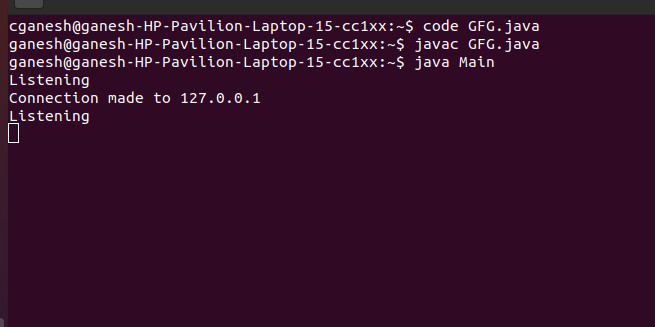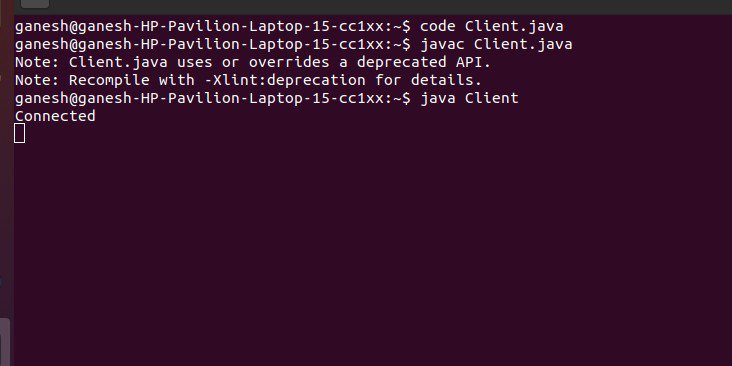GFG App
Open AppBrowser
Continue

# How to Make a Server to Allow the Connection to the Socket 6123 in Java?

A socket connection means the two machines have information about each other’s network location (IP Address) and TCP port. The java.net.Socket class represents a Socket. Here, we are going to look at the approach of connecting to socket 6123.

Approach:

• Create an object of Socket class and pass 6123 as an argument.
• Accept connections with accept() method of ServerSocket class.

Server Code:

## Java

 `// Make a server to allow the connection to the socket 6123 ` ` `  `import` `java.io.IOException; ` `import` `java.net.InetAddress; ` `import` `java.net.ServerSocket; ` `import` `java.net.Socket; ` ` `  `public` `class` `Server { ` `    ``public` `static` `void` `main(String[] args) { check(); } ` `    ``private` `static` `void` `check() ` `    ``{ ` `        ``try` `{ ` `            ``// Creating object of ServerSocket class ` `            ``ServerSocket connection ` `                ``= ``new` `ServerSocket(``6123``); ` `           `  `            ``while` `(``true``) { ` `                ``System.out.println(``"Listening"``); ` ` `  `                ``// Creating object of Socket class ` `                ``Socket socket = connection.accept(); ` ` `  `                ``// Creating object of InetAddress class ` `                ``InetAddress address ` `                    ``= socket.getInetAddress(); ` `               `  `                ``System.out.println( ` `                    ``"Connection made to "` `                    ``+ address.getHostAddress()); ` `                ``pause(``1000``); ` `                   `  `                  ``// close the socket ` `                ``socket.close(); ` `            ``} ` `        ``} ` `        ``catch` `(IOException e) { ` `            ``System.out.println(``"Exception detected: "` `+ e); ` `        ``} ` `    ``} ` `    ``private` `static` `void` `pause(``int` `ms) ` `    ``{ ` `        ``try` `{ ` `            ``Thread.sleep(ms); ` `        ``} ` `        ``catch` `(InterruptedException e) { ` `        ``} ` `    ``} ` `}`

OutputClient Code:

## Java

 `// A Java program for a Client ` ` `  `import` `java.net.*; ` `import` `java.io.*; ` ` `  `public` `class` `Client { ` `    ``// initialize socket and input output streams ` `    ``private` `Socket socket = ``null``; ` `    ``private` `DataInputStream input = ``null``; ` `    ``private` `DataOutputStream out = ``null``; ` ` `  `    ``// constructor to put ip address and port ` `    ``public` `Client(String address, ``int` `port) ` `    ``{ ` `        ``// establish a connection ` `        ``try` `{ ` `            ``socket = ``new` `Socket(address, port); ` `            ``System.out.println(``"Connected"``); ` ` `  `            ``// takes input from terminal ` `            ``input = ``new` `DataInputStream(System.in); ` ` `  `            ``// sends output to the socket ` `            ``out = ``new` `DataOutputStream( ` `                ``socket.getOutputStream()); ` `        ``} ` `        ``catch` `(UnknownHostException u) { ` `            ``System.out.println(u); ` `        ``} ` `        ``catch` `(IOException i) { ` `            ``System.out.println(i); ` `        ``} ` ` `  `        ``// string to read message from input ` `        ``String line = ``""``; ` ` `  `        ``// keep reading until "Over" is input ` `        ``while` `(!line.equals(``"Over"``)) { ` `            ``try` `{ ` `                ``line = input.readLine(); ` `                ``out.writeUTF(line); ` `            ``} ` `            ``catch` `(IOException i) { ` `                ``System.out.println(i); ` `            ``} ` `        ``} ` ` `  `        ``// close the connection ` `        ``try` `{ ` `            ``input.close(); ` `            ``out.close(); ` `            ``socket.close(); ` `        ``} ` `        ``catch` `(IOException i) { ` `            ``System.out.println(i); ` `        ``} ` `    ``} ` ` `  `    ``public` `static` `void` `main(String args[]) ` `    ``{ ` `        ``Client client = ``new` `Client(``"127.0.0.1"``, ``6123``); ` `    ``} ` `}`

OutputMy Personal Notes arrow_drop_up